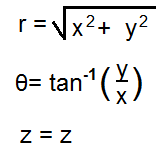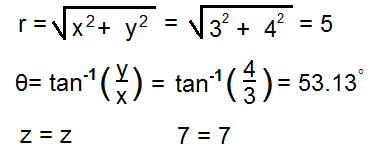﻿ Cartesian to Cylindrical Coordinates Calculator ﻿# Cartesian to Cylindrical Coordinates Calculator### Cartesian (Rectangular) to Cylindrical Coordinates System DiagramX: Y: Z: ° rad

This cylindrical coordinates converter/calculator converts the rectangular (or cartesian) coordinates of a unit to its equivalent value in cylindrical coordinates, according to the formulas shown above.

Rectangular coordinates are depicted by 3 values, (X, Y, Z). When converted into cylindrical coordinates, the new values will be depicted as (r, θ, z).

To use this calculator, a user just enters in the (X, Y, Z) values of the rectangular coordinates and then clicks the 'Calculate' button, and the cylindrical coordinates will be automatically computed and shown below.

This calculator can be used to convert 2-dimensional (2D) or 3-dimensional cartesian coordinates to its equivalent cylindrical coordinates. If desired to convert a 2D cartesian coordinate, then the user just enters values into the X and Y form fields and leaves the 3rd field, the Z field, blank. Z will will then have a value of 0. If desired to convert a 3D cartesian coordinate, then the user enters values into all 3 form fields, X, Y, and Z.

By default, the calculator will compute the result in degrees. However, by using the drop-down menu, the option can changed to radians, so that the result can also be computed in radians.

Example Calculations
Convert the rectangular coordinates (3, 4, 5) into its equivalent cylindrical coordinates.This answer is calculated in degrees. In radians, the value of θ would be 0.93.

Related Resources

﻿